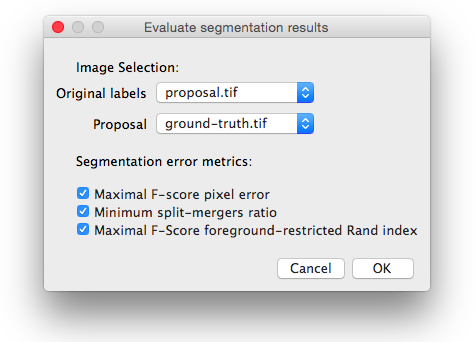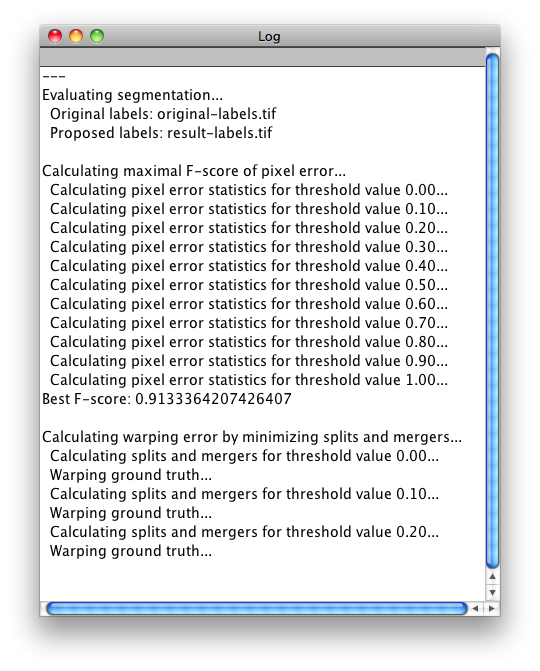# Segmentation evaluation metrics - ScriptThe content of this page has not been vetted since shifting away from MediaWiki. If you’d like to help, check out the how to help guide!

The following Beanshell script allows you to evaluate the performance of your segmentation method.

Just copy/paste it in the Script Editor or save it into a .bsh file and run it (FileOpen):

``````/**
* Script to calculate the segmentation error between some 2D
* original labels and their corresponding proposed labels.
*
* The evaluation metrics are:
*  - Pixel error: 1 - maximal F-score of pixel similarity
*  - Minimum Splits & Mergers Warping error
*  - Foreground-restricted Rand error: 1 - maximal F-score of
*    foreground-restricted Rand index
*
* @author Ignacio Arganda-Carreras (iarganda@mit.edu)
* @version January 22, 2015
*/

import trainableSegmentation.metrics.*;
import ij.WindowManager;
import ij.gui.GenericDialog;
import ij.IJ;

// Get the list of images that are open
ids = WindowManager.getIDList();

if ( ids == null || ids.length < 2 )
{
IJ.showMessage( "You should have at least two images open." );
return;
}

// Get all the titles of the open images
titles = new String[ ids.length ];
for ( int i = 0; i < ids.length; ++i )
{
titles[ i ] = ( WindowManager.getImage( ids[ i ] ) ).getTitle();
}

// Create dialog
gd = new GenericDialog( "Evaluate segmentation results" );

gd.addMessage( "Image Selection:" );
current = WindowManager.getCurrentImage().getTitle();
gd.addChoice( "Original_labels", titles, current );
gd.addChoice( "Proposal", titles, current.equals( titles[ 0 ] ) ? titles[ 1 ] : titles[ 0 ] );

gd.addMessage( "Segmentation error metrics:" );
gd.addCheckbox( "Maximal F-score pixel_error", true );
gd.addCheckbox( "Minimum split-mergers ratio", true );
gd.addCheckbox( "Maximal F-Score foreground-restricted Rand index", true );

gd.showDialog();

if (gd.wasCanceled())
return;

originalLabels = WindowManager.getImage( ids[ gd.getNextChoiceIndex() ] );
proposedLabels = WindowManager.getImage( ids[ gd.getNextChoiceIndex() ] );

calculatePixelError = gd.getNextBoolean();
calculateWarpingError = gd.getNextBoolean();
calculateRandError = gd.getNextBoolean();

IJ.log("---");
IJ.log("Evaluating segmentation...");
IJ.log("  Original labels: " + originalLabels.getTitle());
IJ.log("  Proposed labels: " + proposedLabels.getTitle() + "\n");

// Calculate segmentation error with the selected metrics

if( calculatePixelError )
{
IJ.log("\nCalculating pixel error...");
metric = new PixelError( originalLabels, proposedLabels );
maxFScore = metric.getPixelErrorMaximalFScore( 0.0, 1.0, 0.1 );
IJ.log("  Minimum pixel error: " + (1.0 - maxFScore) );
}

if( calculateWarpingError )
{
IJ.log("\nCalculating warping error by minimizing splits and mergers...");
metric = new WarpingError( originalLabels, proposedLabels );
warpingError = metric.getMinimumSplitsAndMergersErrorValue( 0.0, 0.9, 0.1, false, 20 );
IJ.log("  Minimum warping error: " + warpingError);
IJ.log("  # errors (splits + mergers pixels) = " + Math.round(warpingError * originalLabels.getWidth() * originalLabels.getHeight() * originalLabels.getImageStackSize() ) );
}

if( calculateRandError )
{
IJ.log("\nCalculating maximal F-score of the foreground-restricted Rand index...");
metric = new RandError( originalLabels, proposedLabels );
maxFScore = metric.getForegroundRestrictedRandIndexMaximalFScore( 0.0, 1.0, 0.1 );
IJ.log("  Minimum foreground-restricted Rand error: " + (1.0 - maxFScore) );
}
``````

If you run it, the following dialog will pop up:Here you can select among the open images which ones are the original and the proposed labels, along with the specific metrics you want to apply to evaluate the segmentation results.

After clicking OK the metrics will be applied and the results will be shown in the Log window: